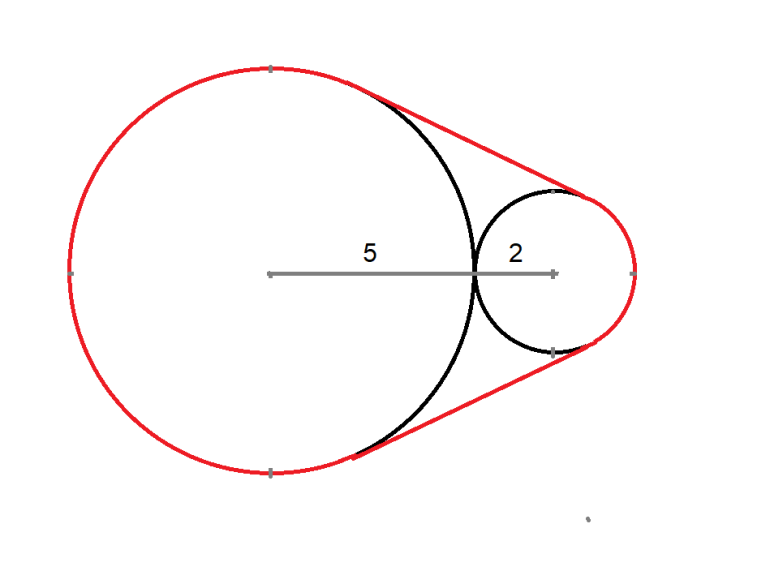## Wizard Recommends

It takes Alice and Bill 3 hours to paint a house.
It takes Alice and Cindy 4 hours to paint a house.
It takes Bill and Cindy 5 hours to paint a house.

How long does it take if they all paint?

"Anonymous" .

120/47 =~ 2.553191 hours

Here is my solution (PDF).

A rubber band is stretched over two adjacent circles of diameters 2 and 5. What is the length of the stretched rubber band?"Anonymous" .

2*sqrt(40) + 10*(π - cos-1(3/7)) =~ 37.297725

Here is my solution (PDF).

This problem is asked and discussed in my forum at Wizard of Vegas.

Consider a game of rock-paper-scissors where:

• If rock beats scissors, scissors pays rock \$1
• If scissors beats paper, paper pays scissors \$2
• If paper beats rock, rock pays paper \$3
• No money changes hands on a tie

Assume two logicians are playing. What is the optimal strategy for each?

"Anonymous" .

• Play paper with probability 1/6
• Play rock with probability 1/3
• Play scissors with probability 1/2

Let's define some probabilities for the logician seeking to maximize his winnings:

• r = Probability of playing rock
• p = Probability of playing paper
• s = Probability of playing scissors

It's clear that r+p+s = 1. Given r and p, s = 1-r-p.

If two logician's play, then both would be indifferent to what their opponent plays, assuming they randomize their choice.

The expected win of the opponent play rock is: 3p-(1-r-p) = 4p+r-1

The expected win of the opponent play paper is: 2(1-r-p) - 3r = 2-5r-2p

The expected win of the opponent play scissors is: r - 2p

These three equations should all be equal.

Setting the first and third equal to each other we get:

4p+r-1 = r - 2p

p = 1/6

Setting the first and second equal to each other we get:

2 -5r - 2p = 4p + r -1